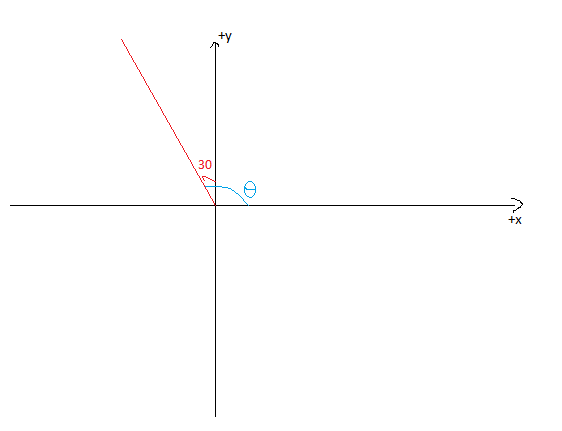Questions & AnswersQuestionAnswers

# Find the slope of the line, which makes an angle of $30^\circ$ with the positive direction of y-axis measured anticlockwise.Answer Verified
Hint: Need to visualize the given information on coordinate axes. Slope of a line is measured with the help of the angle measured from the line with respect to the positive x-axis.

Complete step-by-step answer:
Given that a line makes an angle of $30^\circ$ with the positive y-axis measured anti clockwise.

That means the corresponding figure will be like,We need to find the $\theta$, which is measured from the line with respect to positive x-axis.

Then $\theta = 30^\circ + 90^\circ = 120^\circ$
Thus, slope of the given line is $\tan \theta = \tan 120^\circ$
$\Rightarrow \tan 180^\circ = \tan (180^\circ - 60^\circ ) = - \tan 60^\circ = - \sqrt 3$
$\therefore$ The slope of the given line is $- \sqrt 3$.

Note: To find the slope of a line, we find the inclination angle and apply a tangent to that angle to give the slope. Inclination angle is the angle measured with positive x-axis and the line. We need to know the basic trigonometric function values to solve these kinds of problems.
We used values: $\tan (180 - \theta ) = - \tan \theta$, $\tan 60^\circ = \sqrt 3$.
Bookmark added to your notes.
View Notes
Line Equation Point SlopeCauses of Downward SlopeDifferent Forms of the Equation of LineThe Angle Between Two LinesAn Overview of the Types of ComputerSlope FormulaDeterminant to Find the Area of a TriangleAngle Between a Line and a PlaneThe Language of MathematicsHow to Find The Median?